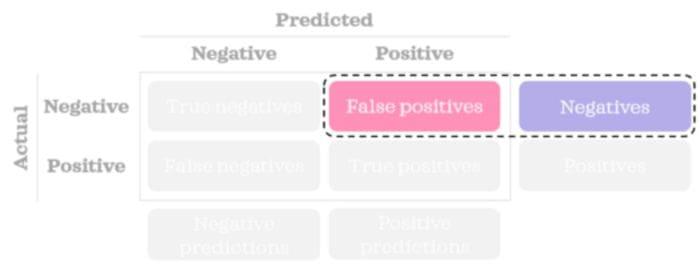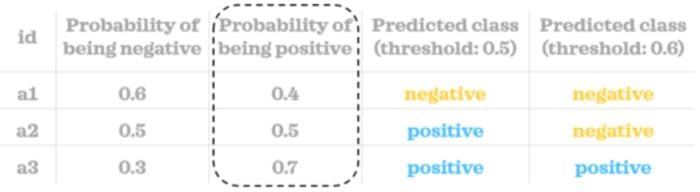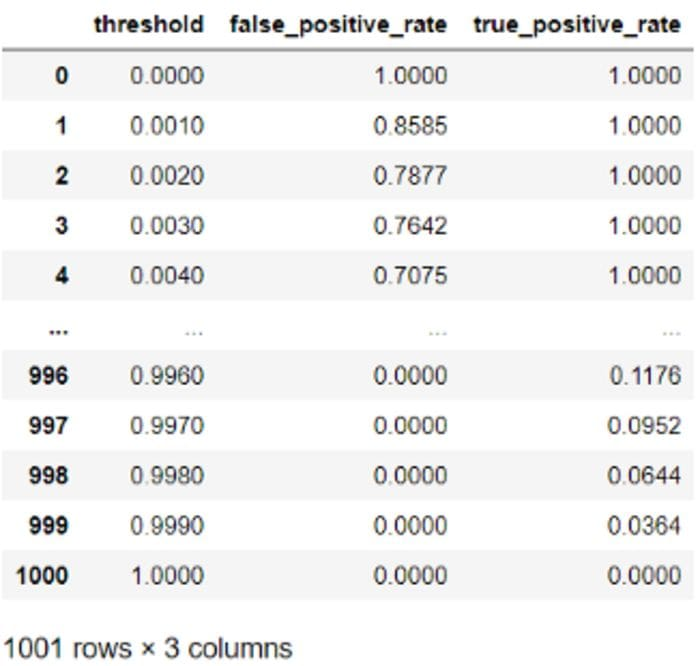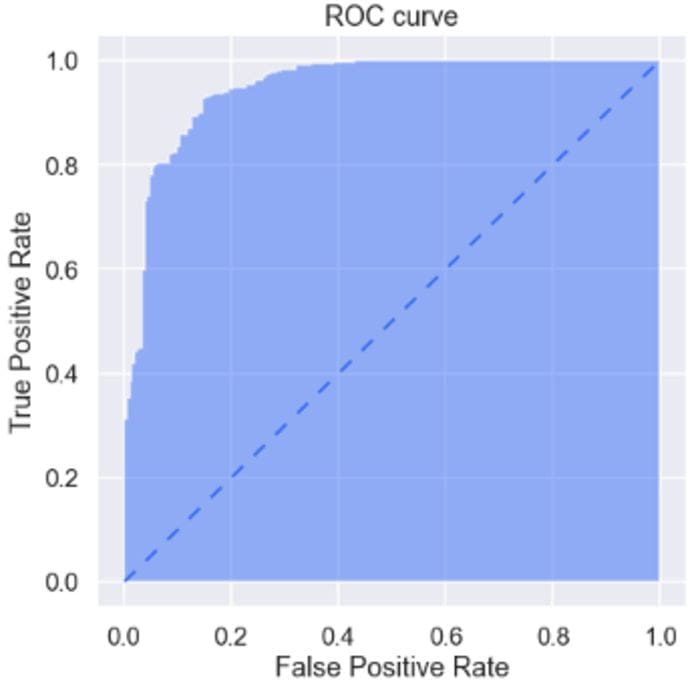# ROC Curve Explained

Learn to visualise a ROC curve in Python.

By Zolzaya Luvsandorj, Data Scientist at iSelect

Area under the ROC curve is one of the most useful metrics to evaluate a supervised classification model. This metric is commonly referred to as ROC-AUC. Here, the ROC stands for Receiver Operating Characteristic and AUC stands for Area Under the Curve. In my opinion, AUROCC is a more accurate abbreviation but perhaps doesn’t sound as nice. In the right context, AUC can also imply ROC-AUC even though it can refer to area under any curve.Photo by Joel Filipe on Unsplash

In this post, we will understand how the ROC curve is constructed conceptually, and visualise the curve in a static and interactive format in Python.

### Understanding the curve

A ROC curve shows us the relationship between False Positive Rate (aka FPR) and True Positive Rate (aka TPR) across different thresholds. Let’s understand what each of these three terms mean.

Firstly, let’s start with a refresher on how a confusion matrix looks like:Image by author

Having refreshed our memory on confusion matrix, let’s look at the terms.

### False Positive Rate

We can find the FPR using the simple formula below:FPR tells us the percentage of incorrectly predicted negative records.Image by author

### True Positive Rate

We can find the TPR using the simple formula below:TPR tells us the percentage of correctly predicted positive records. This is also known as Recall or Sensitivity.Image by author

### Threshold

In general, a classification model can predict the probability of being a certain class for a given record. By comparing the probability value to a threshold value we set, we can classify the record into a class. In other words, you will need to define a rule similar to the following:

If the probability of being positive is greater than or equal to the threshold, then a record is classified as a positive prediction; otherwise, a negative prediction.

In the small example below, we can see the probability scores for three records. Using two different threshold values (0.5 and 0.6), we classified each record into a class. As you can see, the predicted classes vary depending on the threshold value we choose.Image by author

When building a confusion matrix and calculating rates like FPR and TPR, we need predicted classes rather than probability scores.

### ROC curve

Now that we know what FPR, TPR and threshold values are, it’s easy to understand what a ROC curve shows. When constructing the curve, we first calculate FPR and TPR across many threshold values. Once we have the FPR and TPR for the thresholds, we then plot FPR on the x-axis and TPR on the y-axis to get a ROC curve. That’s it! ✨Image by author

Area under a ROC curve ranges from 0 to 1. A completely random model has an AUROCC of 0.5 which is represented by the dashed blue triangle diagonal line below. The further the ROC curve is from this line, the more predictive the model is.Image by author

Now, it’s time to look at some code examples to consolidate our knowledge.

### Build static ROC curve in Python

Let’s first import the libraries that we need for the rest of this post:

``````import numpy as np
import pandas as pd
pd.options.display.float_format = "{:.4f}".formatfrom sklearn.datasets import load_breast_cancer
from sklearn.linear_model import LogisticRegression
from sklearn.metrics import roc_curve, plot_roc_curveimport matplotlib.pyplot as plt
import seaborn as sns
import plotly.express as px
sns.set(palette='rainbow', context='talk')``````

Now we will build a function that will find us the number of false positives and true positives given the correct class, predicted probability of being a positive class and a threshold:

``````def get_fp_tp(y, proba, threshold):
"""Return the number of false positives and true positives."""
# Classify into classes
pred = pd.Series(np.where(proba>=threshold, 1, 0),
dtype='category')
pred.cat.set_categories([0,1], inplace=True)
# Create confusion matrix
confusion_matrix = pred.groupby([y, pred]).size().unstack()\
.rename(columns={0: 'pred_0',
1: 'pred_1'},
index={0: 'actual_0',
1: 'actual_1'})
false_positives = confusion_matrix.loc['actual_0', 'pred_1']
true_positives = confusion_matrix.loc['actual_1', 'pred_1']
return false_positives, true_positives``````

Please note that you will be working with partitioned data sets (e.g. training, test) in reality. But we will not partition our data for simplicity in this post.

We will build a simple model on a toy dataset and get the probabilities of being positive (represented by a value of 1) for the records:

``````# Load sample data
X = load_breast_cancer()['data'][:,:2] # first two columns only
y = load_breast_cancer()['target']# Train a model
log = LogisticRegression()
log.fit(X, y)# Predict probability
proba = log.predict_proba(X)[:,1]``````

We will use 1001 different thresholds between 0 and 1 with increments of 0.001. In other words, threshold values will look something like 0, 0.001, 0.002, … 0.998, 0.999, 1. Let’s find the FPR and TPR for the threshold values.

``````# Find fpr & tpr for thresholds
negatives = np.sum(y==0)
positives = np.sum(y==1)columns = ['threshold', 'false_positive_rate', 'true_positive_rate']
inputs = pd.DataFrame(columns=columns, dtype=np.number)
thresholds = np.linspace(0, 1, 1001)for i, threshold in enumerate(thresholds):
inputs.loc[i, 'threshold'] = threshold
false_positives, true_positives = get_fp_tp(y, proba, threshold)
inputs.loc[i, 'false_positive_rate'] = false_positives/negatives
inputs.loc[i, 'true_positive_rate'] = true_positives/positives
inputs``````Data for the plot is ready. Let’s plot it:

``````def plot_static_roc_curve(fpr, tpr):
plt.figure(figsize=[7,7])
plt.fill_between(fpr, tpr, alpha=.5)
# Add dashed line with a slope of 1
plt.plot([0,1], [0,1], linestyle=(0, (5, 5)), linewidth=2)
plt.xlabel("False Positive Rate")
plt.ylabel("True Positive Rate")
plt.title("ROC curve");

plot_static_roc_curve(inputs['false_positive_rate'],
inputs['true_positive_rate'])``````While building a custom function helps us understand the curve and its inputs, and control them better, we can also take advantage of sklearn’s capabilities that are more optimised. For instance, we can get FPR, TPR and thresholds with a `roc_curve()` function. We can plot the data the same way using our custom plotting function:

``````fpr, tpr, thresholds = roc_curve(y, proba)
plot_static_roc_curve(fpr, tpr)``````Sklearn also provides a `plot_roc_curve()` function which does all the work for us. All you need is a single line (adding title is optional):

``````plot_roc_curve(log, X, y)
plt.title("ROC curve"); # Add a title for clarity``````### Plot interactive ROC curve in Python

When using static plots, it’s hard to see the corresponding threshold value for different points across the curve. One option is to inspect the `inputs `dataframe we created. Another option is to create an interactive version of the plot so that we can see the FPR and TPR alongside the corresponding threshold value when we hover over the graph:

``````def plot_interactive_roc_curve(df, fpr, tpr, thresholds):
fig = px.area(
data_frame=df,
x=fpr,
y=tpr,
hover_data=thresholds,
title='ROC Curve'
)
fig.update_layout(
autosize=False,
width=500,
height=500,
title_x=.5, # Centre title
hovermode = 'closest',
xaxis=dict(hoverformat='.4f'),
yaxis=dict(hoverformat='.4f')
)
hovertemplate = 'False Positive Rate=%{x}<br>True Positive Rate=%{y}<br>Threshold=%{customdata:.4f}<extra></extra>'
fig.update_traces(hovertemplate=hovertemplate)

# Add dashed line with a slope of 1
fig.add_shape(type='line', line=dict(dash='dash'), x0=0, x1=1, y0=0, y1=1)
fig.show()plot_interactive_roc_curve(df=inputs,
fpr='false_positive_rate',
tpr='true_positive_rate',
thresholds=['threshold'])``````The interactivity is quite useful, isn’t it?

Hope you enjoyed learning how to build and visualise a ROC curve. Once you understand this curve, it’s easy to understand another related curve: Precision-Recall curve.

Bye for now 🏃💨

Bio: Zolzaya Luvsandorj works as a Data Scientist at iSelect. Upon completing her BCom as a top student with multiple prestigious awards, Zolzaya worked as a Data Analyst in a consultancy firm for 3 years before moving on to her current role. She loves expanding her knowledge in data science, computer science and statistics and explaining data science concepts in simple words in her blogs.

Original. Reposted with permission.

Related: